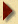### Gas Compressibility Factor "Z" - Redlich-Kwong Method

The Redlich-Kwong generalised equation of state provides a simple method for estimating the compressibility factor, Z, of real gases. While the method is not accurate enough for royalty metering applications, it is plainly sufficient for sizing valves or other problems where an estimate of the gas density is required. Typically, Z is estimated to +/- 5%.

Z calculator

 Critical Pressure kPa (abs) Molecular weight Critical Temperature K Flowing Density (kg/m3) Operating Pressure kPa (gauge) Normal Density (kg/m3) Operating Temperature °C Standard Density (kg/m3) Z

Normal conditions are 0°C and 101.325 kPa
Standard conditions are 60°F and 14.695 psiReturn